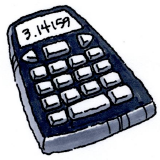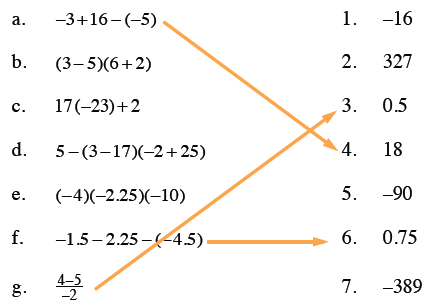Home > ACC7 > Chapter cc32 > Lesson cc32.1.7 > Problem2-71

2-71.

CALCULATOR CHECK

Use your scientific calculator to compute the value of each expression in the left-hand column below. Match each result to an answer in the right-hand column. Homework Help ✎1. $−3+16−(−5)$

2. $(3−5)(6+2)$

3. $17(−23)+2$

4. $5−(3−17)(−2+25)$

5. $(−4)(−2.25)(−10)$

6. $−1.5−2.25−(−4.5)$

7. $\frac{4-5}{-2}$

1. $−16$

1. $327$

2. $0.5$

3. $18$

4. $−90$

5. $0.75$

6. $−389$

Remember to be cautious when entering negative signs.
A few matches have been made below, so that you can check some of your work.